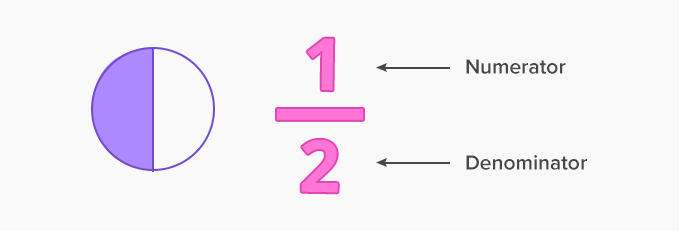# Simplifying Fractions - Definition with Examples

The Complete K-5 Math Learning Program Built for Your Child

• 40 Million Kids

Loved by kids and parent worldwide

• 50,000 Schools

Trusted by teachers across schools

• Comprehensive Curriculum

Aligned to Common Core

Simplifying fractions

A fraction is a part of a whole. It has a numerator and a denominator.

For example: 1here 1 is the numerator and 2 is the denominator. It is represented by the figure given below:Here, the shape is divided into 2 equal parts, therefore the denominator is 2 and 1 out of 2 parts is shaded, therefore the numerator is 1.

Simplest form of a fraction

A fraction is said to be in its simplest form if 1 is the only common factor of its numerator and denominator. For example,89,because 1 is the only common factor of 8 and 9 in this fraction.

We simplify fractions because it is always to work or calculate when the fractions are in the simplest form.

Simplifying proper and improper fractions

Steps:

Find the highest common factor (HCF) of the numerator and denominator.

Divide both the numerator and denominator by HCF.

Example 1: Check if the fraction 715 is in its simplest form?

Solution: Factors of numerator 7 = 1, 7

Factors of denominator 15 = 1, 3, 5, 15

We can see that 1 is the only common factor of 7 and 15

Therefore, 715 in its simplest form.

Example 2: Reduce 1218 in its simplest form.

Solution: Factors of 12 = 1, 2, 3, 4, 6, 12

Factors of 18 = 1, 2, 3, 6, 9, 18

Highest common factor (HCF) of 12 and 18 = 6

Dividing the numerator and the denominator by 6 (HCF), we get

12÷6 18÷6 = 2 3

Hence,  2is the simplest form of the fraction 1218.

Simplifying a mixed fraction

Steps:

• Find the highest common factor (HCF) of numerator and denominator of the fraction.
• Divide both the numerator and the denominator by HCF to get the simplified fraction.
• Write the whole and the simplified fraction together.

Example 4: Matthew has31216of ice cream. How much ice cream does he have in its simplest form?

Solution: Factors of 3 12 16 = 1 4 12 = 1, 2, 3, 4, 6, 12

Factors of 16 = 1, 2, 4, 8, 16

Highest common factor (HCF) of 12 and 16 = 4

Dividing the numerator and denominator by 4 (HCF), we get

12÷4 16÷4 = 3 4

Hence, Matthew has 31216of ice cream with him in its simplest form.

 Fun Facts: The word fraction comes from the Latin word ‘Fractio’ which means to break. Every whole number can be written as a fraction. For example, 2 can be written as 21

Won Numerous Awards & Honors